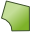# PH Card

The PH card creates a triangular or quadrangular plate with a circular or elliptical hole as shown in the card. The hole can be used, for example, to attach a cylinder (ZY card) to the plate and it can be filled with the KR card.

On the Construct tab, in the Surfaces group, click thePlate with hole (PH) icon.

## Parameters:

S1
The corner where the hole is located (also the hole's centre).
S2
The second corner of the plate.
S3
The third corner of the plate. If this field is left empty, a triangular plate is created.
S4
The fourth corner of the plate.
S5
A point on the line S1–S2 that forms the starting point of the circle or ellipse bordering the hole. The special case where S5 is identical to S2 is supported, but due to the resulting geometry yields triangles with very small angles.
Max. edge length on curve
The maximum edge length of the triangles along the edge of the hole in m (is scaled by the SF card). If this parameter is left empty, the value specified with the IP card is used.
Scale second half axis with
If this parameter is empty or is set to 1, a circular hole is created. If set to $\frac{b}{a}$ , an elliptical hole is created. Here $\frac{b}{a}$ gives the ratio of the two half axes, where $a$ is the distance S1–S3. It is not recommended to generate the plate with a CAD system if the elliptical hole has an extremely small or extremely large axial ratio as the distortion formulation used in PREFEKO may fail for such cases.

## Examples of PH card usage:

The PH card can be used to create the rectangular plate shown in Figure 2.

Note the extremely narrow triangles at the corners, as shown in Figure 3 and Figure 4, for a quadrangular and rectangular plate with the same elliptical hole. The triangular plate is obtained by leaving the field S3 empty.